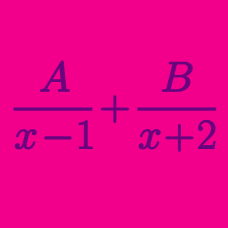Algebra

Partial Fractions - Cover Up Rule

By the coverup rule, the coefficient of the term $\frac{1}{ x + 6 }$ in the partial fraction decomposition of

$\frac{ 1} { ( x + 6 ) ( x + 19 ) },$

has the form $\frac{1}{A}$. What is $A$?

By the coverup rule, the coefficient of the term $\frac{1}{ x + 2 }$ in the partial fraction decomposition of

$\frac{ 1} { ( x - 2 ) ( x + 2 ) ( x + 7 ) },$

has the form $\frac{1}{A}$. What is $A$?

By the coverup rule, the coefficient of the term $\frac{1}{ x + 4 }$ in the partial fraction decomposition of

$\frac{ 1} { ( x + 4 ) ( x^2 + 5 x + 20 ) },$

has the form $\frac{1}{A}$. What is $A$?

By the coverup rule, the coefficient of the term $\frac{1}{ x + 2 }$ in the partial fraction decomposition of

$\frac{ 1} { ( x + 2 ) ( x^2 + 4 x + 28 ) },$

has the form $\frac{1}{A}$. What is $A$?

By the coverup rule, the coefficient of the term $\frac{1}{ x + 3 }$ in the partial fraction decomposition of

$\frac{ 1} { ( x + 3 ) ( x + 9 )(x+16) },$

has the form $\frac{1}{A}$. What is $A$?

×